# SBI PO Prelims Quantitative Aptitude Questions 2019 – Day 12

SBI PO 2019 Notification will be expected soon. It is one of the most expected recruitment among the banking aspirants. Every year the exam pattern for SBI PO has been changing. Depends upon the changing of exam pattern the questions are quite harder compare to the previous year. So the questions are in high level than the candidate’s assumption.

As per the latest trend, our IBPS Guide is providing the updated New Exam Pattern Quantitative Aptitude questions for SBI PO 2019 Day 12. Our Skilled experts were mounting the questions based on the aspirant’s needs. So candidates shall start your preparation and practice on daily basis with our SBI PO pattern quantitative aptitude questions 2019 day 12. Start your effective preparation from the right beginning to get success in upcoming SBI PO 2019.

[WpProQuiz 5175]

### Click Here for SBI PO Pre 2019 High-Quality Mocks Exactly on SBI Standard

Directions (1 – 5): The questions below are based on the given Series-I. The series-I satisfy a certain pattern, follow the same pattern in Series-II and answer the questions given below.

1) I) 12, 14, 31, 97, 393, 1971

II) 75 ………. 9531. If 9531 is nth term, then find the value of n?

a) 3rd term

b) 4th term

c) 5th term

d) 6th term

e) None of these

2) I) 72, 110, 158, 223, 314, 442

II) 35, 77 ………. 607. If 607 is nth term, then find the value of n?

a) 7th term

b) 6th term

c) 5th term

d) 4th term

e) None of these

3) I) 24, 144, 720, 2880, 8640, 17280

II) 42 ……. 30240. If 30240 is nth term, then find the value of n?

a) 6th term

b) 5th term

c) 7th term

d) Both 6th and 7th term

e) None of these

4) I) 792, 396, 264, 198, 158.4

II) 594 …….. 99. If 99 is nth term, then find the value of n?

a) 4th term

b) 6th term

c) 5th term

d) 7th term

e) None of these

5) I) 36, 292, 420, 484, 516, 532

II) 18, 234 …….. 423. If 423 is nth term, then find the value of n?

a) 4th term

b) 7th term

c) 5th term

d) 6th term

e) None of these

Directions (Q. 6 – 10): Study the following information carefully and answer the given questions:

The following table shows the total number of terrorist attacks registered in 5 different countries in 5 different years.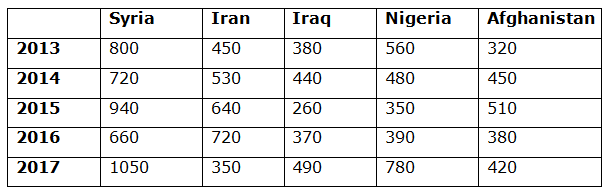6) Find the total number of terrorist attacks registered in first half of the year in Syria and Nigeria in the year 2013 and 2015 respectively?

Statement I: The percentage of terrorist attacks registered in first half of the year in Syria in the year 2013 is 42 %.

Statement II: Total number of terrorist attacks registered in last half of the year in Syria and Nigeria in the year 2013 and 2015 together is 52 % of total number of terrorist attacks registered in both the countries in the year 2013 and 2015.

a) Only I

b) Both I and II

c) Only II

d) Neither I nor II

e) Either I or II

7) Find the difference between the total number of terrorist attacks registered in Iran to that of Nigeria in all the given years together?

a) 220

b) 180

c) 130

d) 90

e) None of these

8) Total number of terrorist attacks registered in the year 2013 is approximately what percentage of total number of terrorist attacks registered in the year 2015 in all the given countries together?

a) 93 %

b) 108 %

c) 85 %

d) 77 %

e) 62 %

9) Quantity I: Find the average number of terrorist attacks registered in the year 2016?

Quantity II: Find the difference between the average number of terrorist attacks registered in Syria to that of Afghanistan in all the given years together?

a) Quantity I > Quantity II

b) Quantity I < Quantity II

c) Quantity I ≤ Quantity II

d) Quantity I ≥ Quantity II

e) Quantity I = Quantity II

10) Total number of terrorist attacks registered in Iraq in all the given years together is approximately what percentage more/less than the total number of terrorist attacks registered in the year 2015 of all the given countries together?

a) 15 % less

b) 28 % more

c) 42 % less

d) 28 % less

e) 15 % more

Direction (1-5 ) :

Series I,

12 is the first term

12*1 + 2 = 14

14*2 + 3 = 31

31*3 + 4 = 97

97*4 + 5 = 393

393*5 + 6 = 1971

1971 is 6th term

Series II,

75 is the first term

75*1 + 2 = 77

77*2 + 3 = 157

157*3 + 4 = 475

475*4 + 5 = 1905

1905*5 + 6 = 9531

9531 is 6th term

Series I,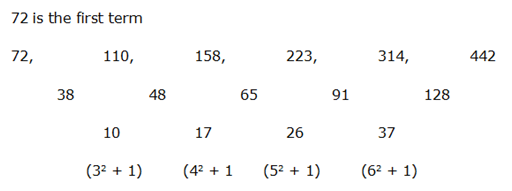442 is 6th term.

Series II,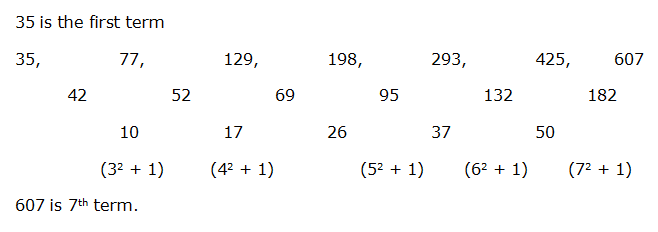3) Answer: d)

Series I,

24 is the first term

24*6 = 144

144*5 = 720

720*4 = 2880

2880*3 = 8640

8640*2 = 17280

17280 * 1 = 17280

17280 is 6th term. 7th term also 17280. So, both 6th and 7th term.

Series II,

42 is the first term

42*6 = 252

252*5 = 1260

1260*4 = 5040

5040*3 = 15120

15120*2 = 30240

30240*1 = 30240

Both 6th and 7th term

Series I,

792 is the first term

792*(1/2) = 396

396*(2/3) = 264

264*(3/4) = 198

198*(4/5) = 158.4

158.4 is the 5th term

Series II,

594 is the first term

594*(1/2) = 297

297*(2/3) = 198

198*(3/4) = 148.5

148.5*(4/5) = 118.8

118.8*(5/6) = 99

99 is the 6th term

Series I,

36 is the first term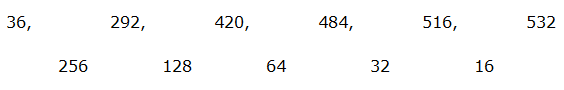The pattern is, the difference of two numbers ÷ 2

532 is 6th term

Series II,

18 is the first term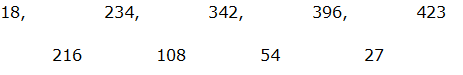423 is 5th term

Direction (6-10) :

The total number of terrorist attacks registered in first half of the year in Syria and Nigeria in the year 2013 and 2015 respectively

From Statement I,

Total number of terrorist attacks registered in Syria in the year 2013 = 800

The total number of terrorist attacks registered in first half of the year in Syria in the year 2013

= > 800*(42/100) = 336

Statement I alone is not sufficient to answer the question.

From Statement II,

Total number of terrorist attacks registered in first half of the year in Syria and Nigeria in the year 2013 and 2015 respectively

= > (800 + 350)*(48/100) = 552

Statement II alone is sufficient to answer the question.

The total number of terrorist attacks registered in Iran in all the given years together

= > 450 + 530 + 640 + 720 + 350 = 2690

The total number of terrorist attacks registered in Nigeria in all the given years together

= > 560 + 480 + 350 + 390 + 780 = 2560

Required difference = 2690 – 2560 = 130

Total number of terrorist attacks registered in the year 2013 in all the given countries together

= > 800 + 450 + 380 + 560 + 320 = 2510

Total number of terrorist attacks registered in the year 2015 in all the given countries together

= > 940 + 640 + 260 + 350 + 510 = 2700

Required % = (2510/2700)*100 = 93 %

Quantity I:

The average number of terrorist attacks registered in the year 2016

= > (660 + 720 + 370 + 390 + 380)/5

= > 2520/5 = 504

Quantity II:

The average number of terrorist attacks registered in Syria in all the given years together

= > (800 + 720 + 940 + 660 + 1050)/5 = 834

The average number of terrorist attacks registered in Afghanistan in all the given years together

= > (320 + 450 + 510 + 380 + 420)/5 = 416

Required difference = 834 – 416 = 418

Quantity I > Quantity II

The total number of terrorist attacks registered in Iraq in all the given years together

= > 380 + 440 + 260 + 370 + 490 = 1940

The total number of terrorist attacks registered in the year 2015 of all the given countries together

= > 940 + 640 +260 + 350 + 510 = 2700

Required % = [(2700 – 1940) / 2700]*100 = 28 % less